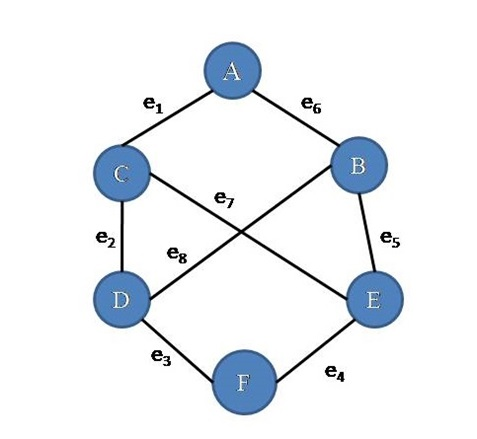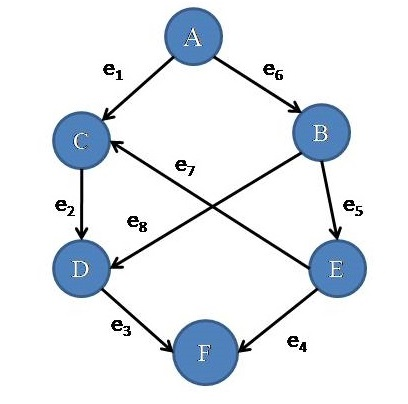# Introduction to Graph in Data Structure

Graph in Data Structure: In this article, we are going to see what is graph data structure and types of graphs?
Submitted by Souvik Saha, on March 17, 2019

What you are going to learn?

• What are the components in Graph?
• Types of the Graphs.
• How we use the Graph data structure?

## Graph

The graph is an abstract data type in computer science. It maps the value between two data nodes.Figure 1.1

### Components

1) Node: In the above example, Graph(Figure 1.1) there is a set of nodes. Nodes basically store values of data types ( int, float etc). In the example A, B, C, D, E, F are the nodes of the graph.

2) Edge: In the example graph, there is a set of edges in a graph. Edges make a relationship between two nodes or they are a connection between nodes in a graph. In the example e1 , e2, e3, e5, e6 ,e7,e8are the edges in that graph. Edges can be weighted or unweighted. In the case of a weighted edge, each edge is assigned a weight.

### Types

There are two types of graphs,

1) Directed Graph: When all the edges have a direction from one node to another node then the graph is called Directed Graph. (Figure 1.2)Figure 1.2

2) Undirected Graph: When all the edges have no direction then it called Undirected Graph. The edges start from one node and go to another node. (Figure 1.1)

Real life Application of graphs:

• Building recommendation system for e-commerce websites

Top MCQs

Languages: » C » C++ » C++ STL » Java » Data Structure » C#.Net » Android » Kotlin » SQL
Web Technologies: » PHP » Python » JavaScript » CSS » Ajax » Node.js » Web programming/HTML
Solved programs: » C » C++ » DS » Java » C#
Aptitude que. & ans.: » C » C++ » Java » DBMS
Interview que. & ans.: » C » Embedded C » Java » SEO » HR
CS Subjects: » CS Basics » O.S. » Networks » DBMS » Embedded Systems » Cloud Computing
» Machine learning » CS Organizations » Linux » DOS
More: » Articles » Puzzles » News/Updates